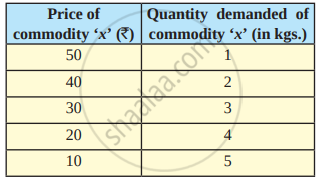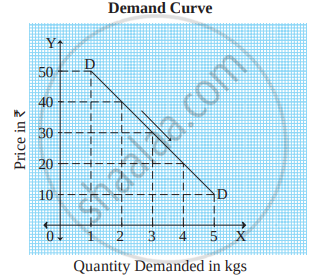# Law of Demand

## Notes

#### Introduction :

The law of demand was introduced by Prof. Alfred Marshall in his book, ‘Principles of Economics’, which was published in 1890. The law explains the functional relationship between price and quantity demanded.

#### Statement of the Law :

According to Prof. Alfred Marshall, “Other things being equal, higher the price of a commodity, smaller is the quantity demanded and lower the price of a commodity, larger is the quantity demanded.” In other words, other factors remaining constant, if the price of a commodity rises, demand for it falls and when price of a commodity falls demand for the commodity rises. Thus, there is an inverse relationship between price and quantity demanded.
Symbolically, the functional relationship between demand and price is expressed as :
Dx = f (Px)

Where D = Demand for a commodity
x = Commodity
f = Function
Px = Price of a commodity

#### Additional Information For better Understanding:

In other words, it can be said that if the price of a commodity reduces additional units of goods can be purchased. Eminent economist like Prof. Marshall has compared this law with a game of “See-Saw” in which he has stated that when one end of a plank of wood goes up the other end goes down.

#### The law of demand is explained with the help of the following demand schedule and diagram:As shown in Table, when price of commodity ‘x’ is  50, quantity demanded is 1 kg. When price falls from  50 to  40, quantity demanded rises from 1 kg to 2 kgs. Similarly, at price  30, quantity demanded is 3 kgs and when price falls from  20 to  10, quantity demanded rises from 4 kg sto 5 kgs
Thus, as the price of a commodity falls,quantity demanded rises and when price of commodity rises, quantity demanded falls. This shows an inverse relationship between price and quantity demanded.In the figure above, X-axis represents the demand for the commodity and Y axis represents the price of commodity x. DD is the demand curve which slopes downward from left to right due to an inverse relationship between price and quantity demanded.

#### Additional Information For better Understanding:

In other words, it can be said that if the price of a commodity reduces additional units of goods can be purchased. Eminent economist like Prof. Marshall has compared this law with a game of “See-Saw” in which he has stated that when one end of a plank of wood goes up the other end goes down.

### For Better Understandng:

This Law of Demand can be expressed more clearly by an example, Suppose, the price of apples per dozen is ₹80, its demand is 1 dozen. When the price is  ₹ 90 then half a dozen,  ₹100 then 4;  ₹110 then two, and so on. From the following table demand of apples can be studied clearly when there is increase and decrease in price.

 Price of apples per dozen Demand for Apples based on the price Conclusion ₹ 80 1 dozen = 12 apples Price ↓ Demand ↑ ₹ 90 Half dozen = 6 apples Price ↑ Demand ↓ ₹ 100 4 apples Price ↑ Demand ↓ ₹ 110 2 apples Price ↑ Demand ↓

If you would like to contribute notes or other learning material, please submit them using the button below.